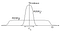# How Bayesian methods embody Occam’s razor

Some of you might have heard the term Occam’s razor, sometimes spelled Ockham’s razor, together with Bayesian methods. It is indeed a great concept which is very useful for many applications. This post is just another argument why Bayesian methods are so widely applicable and must be applied. In a fairly short post, I will explain how Bayesian methods embody Occam’s razor naturally in an intuitive language and directly refer it to deep learning. Much of my post is taken from David MacKay’s PhD thesis (1992). I highly recommend giving it a read.

# Which model performs well for my data set?

Imagine you work in a supervised learning setting, let’s say CIFAR-100, and you are quite new to computer vision, but already know that a convolutional neural network (CNN) is your choice to go. But, you do not really know how large your CNN needs to be to achieve the desired validation accuracy. In fact, even experienced deep learning researchers and practitioners still need to follow some rule of thumbs or trial and error to find an architecture of a CNN which performs well enough when they work with a new data set.

An architecture of a CNN consists of number of hidden layers, filters, activation functions, etc. All of these summarised is meant by the word model.

Comparing multiple models is a difficult task because it is not possible to simply choose the model that fits the data best: more complex models, i.e. more hidden layers and more filters, can always fit the data better. So, the maximum likelihood model choice, i.e. choosing the model with the highest p(D|θ), would lead us inevitably to implausible overparameterised models that generalise poorly. As you know, that’s not what we aim for. Instead, we would like to have a model which generalises well, i.e. it performs well on unseen data examples.

# Occam’s razor

Occam’s razor is the principle that states that unnecessarily complex models should not be preferred to simpler ones.

To clarify, simpler models means less parameters θ to train, thus faster computations and more generalisations. I guess, we agree that all these characteristics are desiderata, particularly in the context of deep learning. Imagine again that we work with CIFAR-100 and hypothetically, the two models in the subsequent figure achieve exactly the same validation accuracy. Why should I waste computational resources to train model b which has more parameters θ to train than model a?

Bayesian methods automatically and quantitatively embody Occam’s razor (Gull, 1988; Jeffreys, 1939). Complex models are automatically self-penalising under Bayes’ rule. Let us review Bayes’ rule to clarify how we came to this conclusion:

This is equivalent to

You can understand H1 as a proposal for a model which is hoped to represent the data D well (H because of hypothesis). In a Bayesian neural network, this hypothesis H1 can be understood as the total set of model parameters θ={θ1, θ2, …, θn}. Let’s assume H1 is a fairly simple model and we have another proposal H2 which is a much larger model. We all agree on that H2 has a much wider range of possible data sets D it can simultaneously perform well on, but the current quest of narrow artificial intelligence is to solve specific tasks, which we will call C1. Specific tasks mean specific data sets. In the subsequent figure, we have a specific data set C1 as a subset of possible data sets D.The degree of evidence for a specific data set C1 as a set of possible data sets D (MacKay, 1992)

The term p(D|H1) is the likelihood for the hypothetical model H1 for the given data D=C1. Recall that we only work with the data set C1. Since we optimise the posterior probability p(H1|D) to be as close to 1 as possible in the given task, Bayes’ rule answers the rhetoric question: “why is it necessary to have a larger model H2?”.

A simple model H1 makes only a limited range of predictions, shown by P(D|H1), whereas a more powerful model H2, that has, for example, more learnable parameters θ than H1, is able to predict a greater variety of data sets. This means, however, that H2 does not predict the data sets in region C1 as strongly as H1. Assume that equal prior probabilities have been assigned to the two models. Then, if the data set falls in region C1, the less powerful model H1 will be the more probable model.

Recall that probabilities are in a Bayesian understanding measures of plausibility. This was put forth by Cox (1946) by seeing all hypothetical solutions to any given problem as a set of which each hypothesis has a certain probability to be the solution to the given problem.

Written by

Written by

## Felix Laumann

#### helping you with the first steps into Bayesian deep learning | PhD Student at Imperial College London | Research Scientist at NeuralSpace